Question

# A disk of uniform mass M and radius R (Icm = 1 / 2MR2) is tied...

A disk of uniform mass M and radius R (Icm = 1 / 2MR2) is tied to a rope that in turn is tied to the ceiling, as shown in figure 2. The mass is released from rest and its center of mass low with a constant acceleration of (Assume that there is friction between the string and the disc and therefore it does not slip.):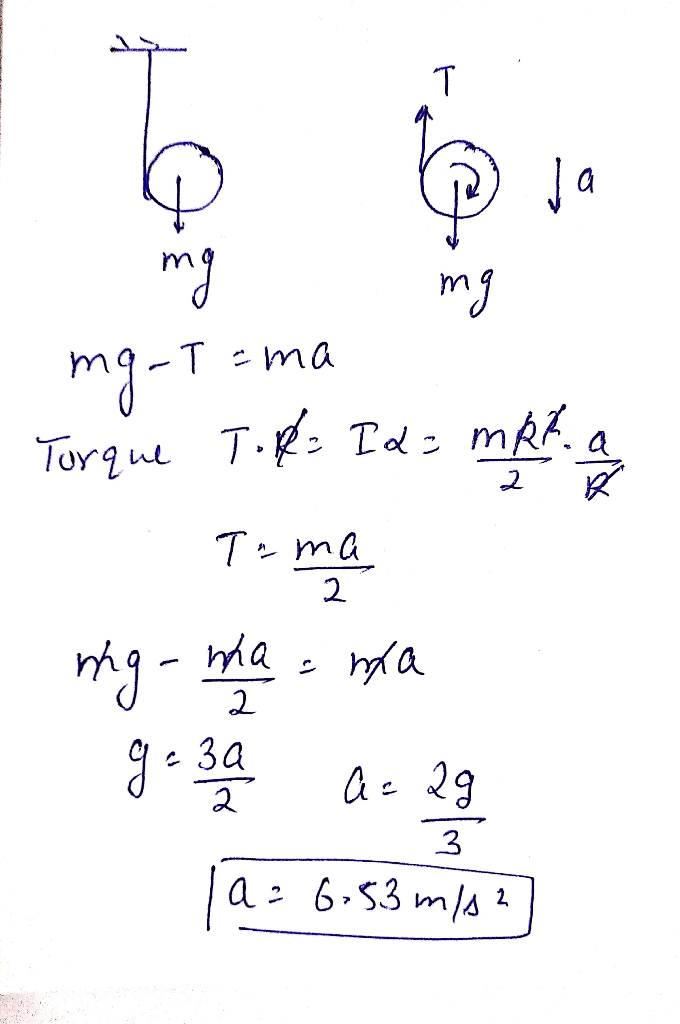#### Earn Coins

Coins can be redeemed for fabulous gifts.

Similar Homework Help Questions
• ### A string is wound around a uniform disk of radius R and mass M. The disk is released from rest with the string vertical and its top end tied to a fixed bar. See the figure below.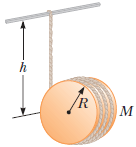A string is wound around a uniform disk of radius R and mass M. The disk is released from rest with the string vertical and its top end tied to a fixed bar. See the figure below. (Submit a file with a maximum size of 1 MB.) (a) Show that the tension in the string is one third of the weight of the disk. (b) Show that the magnitude of the acceleration of the center of mass is 2g/3. (c) Show that the...

• ### A uniform disc with mass M and radius R = 0.10 m is mounted on a...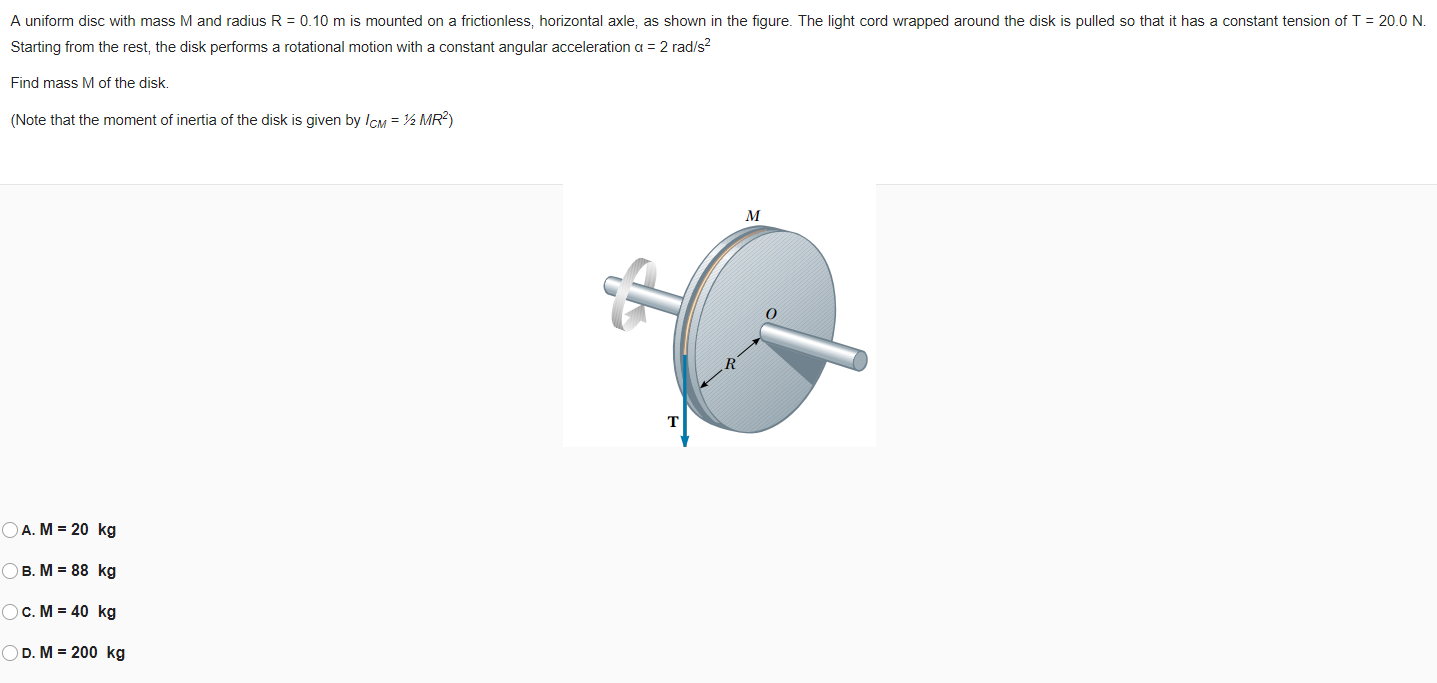A uniform disc with mass M and radius R = 0.10 m is mounted on a frictionless, horizontal axle, as shown in the figure. The light cord wrapped around the disk is pulled so that it has a constant tension of T = 20.0 N. Starting from the rest, the disk performs a rotational motion with a constant angular acceleration a = 2 rad/s2 Find mass M of the disk. (Note that the moment of inertia of the disk is...

• ### Figure 3 Uniform disk Uniform rod 3) Figure 3 illustrates a physical pendulum comprising a uniform disk having mass M and radius R and a rod having the length R and mass M. The disk is pivotally m...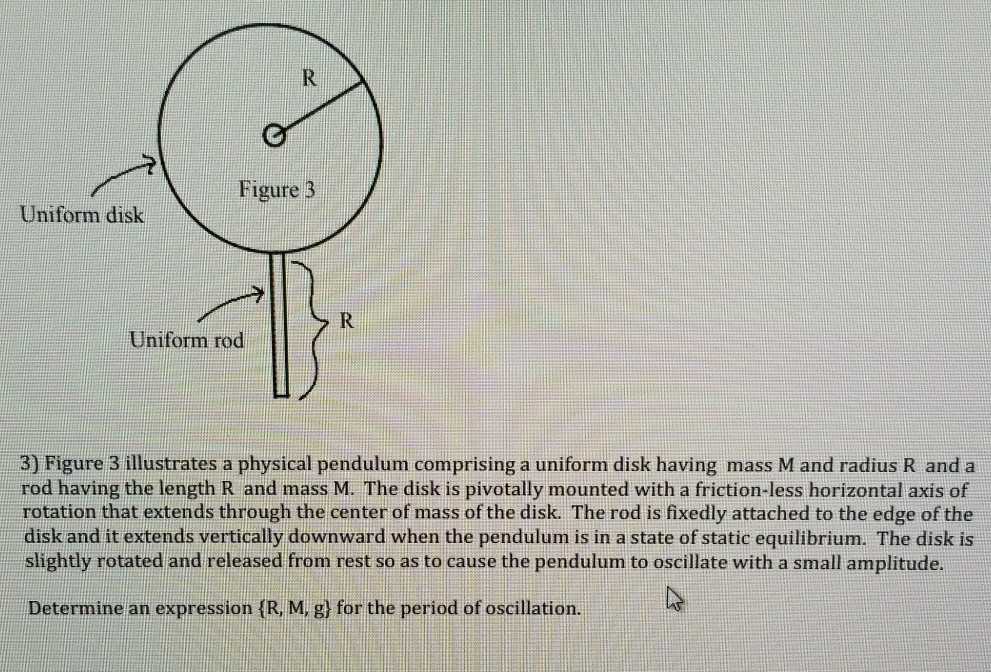Figure 3 Uniform disk Uniform rod 3) Figure 3 illustrates a physical pendulum comprising a uniform disk having mass M and radius R and a rod having the length R and mass M. The disk is pivotally mounted with a friction-less horizontal axis of rotation that extends through the center of mass of the disk. The rod is fixedly attached to the edge of the disk and it extends vertically downward when the pendulum is in a state of static...

• ### 2. The pulley (disk) has a radius "R" and a mass "m". The rope does not...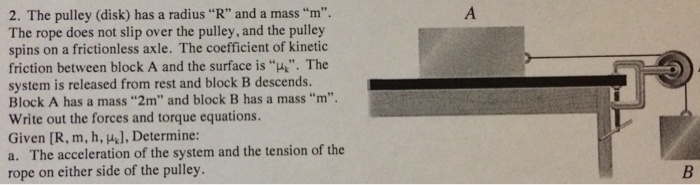2. The pulley (disk) has a radius "R" and a mass "m". The rope does not slip over the pulley, and the pulley spins on a frictionless axle. The coefficient of kinetic friction between block A and the surface is "u. The system is released from rest and block B descends. Block A has a mass "2m" and block B has a mass "m Write out the forces and torque equations. Given [R, m, h, ], Determine: a. The acceleration...

• ### Question 7(a). A string is wound around a uniform disc of mass M and radius R....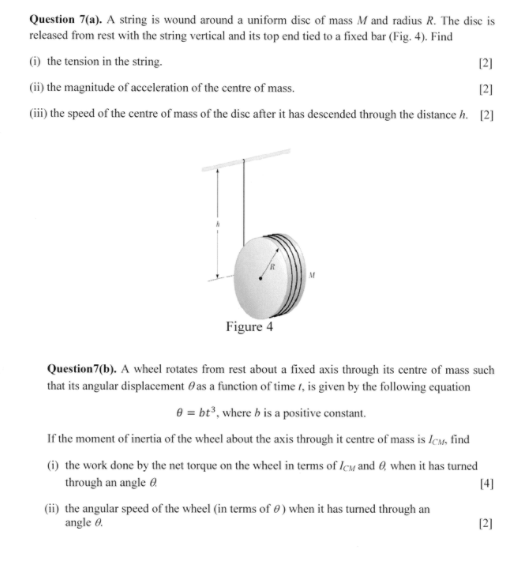Question 7(a). A string is wound around a uniform disc of mass M and radius R. The dise is released from rest with the string vertical and its top end tied to a fixed bar (Fig.4). Find 6 the tension in the string. (ii) the magnitude of acceleration of the centre of mass. (ii) the speed of the centre of mass of the disc after it has descended through the distance h. 2 121 121 Figure 4 Question 7(b). A...

• ### A string is wrapped around a uniform cylinder of mass M and radius R as shown...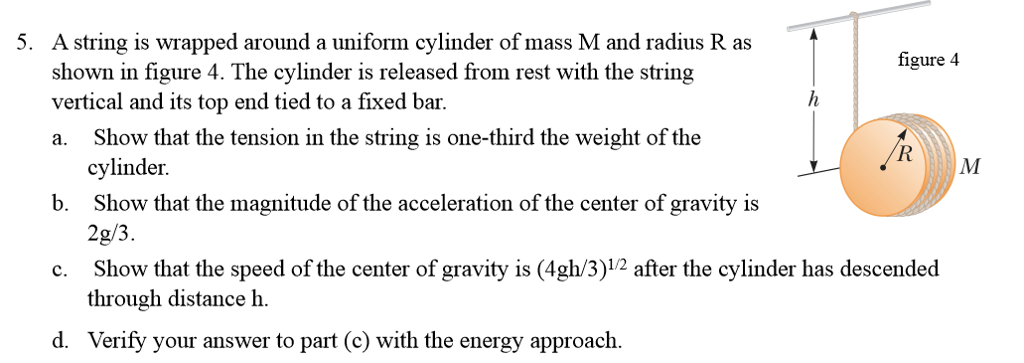A string is wrapped around a uniform cylinder of mass M and radius R as shown in figure 4. The cylinder is released from rest with the string vertical and its top end tied to a fixed bar. a. Show that the tension in the string is one-third the weight of the cylinder. b. Show that the magnitude of the acceleration of the center of gravity is 2g/3. c. Show that the speed of the center of gravity is (4gl/3)^1/2...

• ### A string is wrapped around a uniform disk of mass M = 2.2 kg and radius R = 0.1 m. (Recall that the moment of inertia of a uniform disk is (1/2) MR2.)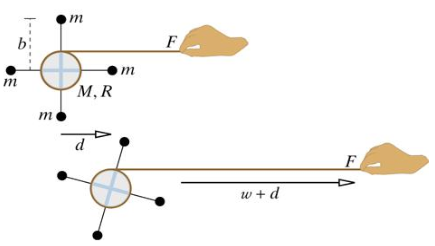A string is wrapped around a uniform disk of mass M = 2.2 kg and radius R = 0.1 m. (Recall that the moment of inertia of a uniform disk is (1/2) MR2.) Attached to the disk are four low-mass rods of radius b = 0.13 m, each with a small mass m = 0.7 kg at the end. The device is initially at rest on a nearly frictionless surface. Then you pull the string with a constant force F...

• ### A uniform, solid cylinder with mass 3M and radius 2R rests on a horizontal tabletop. A...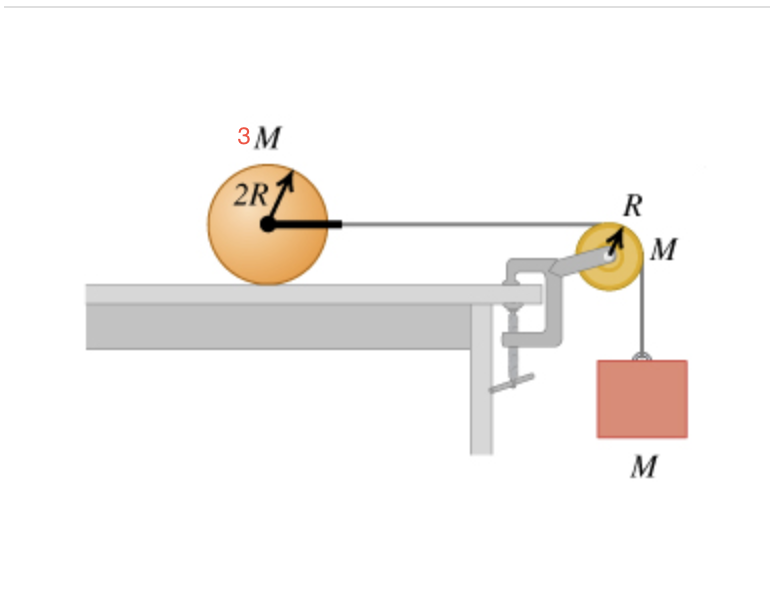A uniform, solid cylinder with mass 3M and radius 2R rests on a horizontal tabletop. A string is attached by a yoke to a frictionless axle through the center of the cylinder so that the cylinder can rotate about the axle. The string runs over a disk-shaped pulley with mass M and radius R that is mounted on a frictionless axle through its center. A block of mass M is suspended from the free end of the string (the figure...

• ### A rod of length L and negligible mass is attached to a uniform disk of mass M and radius R (see figure below).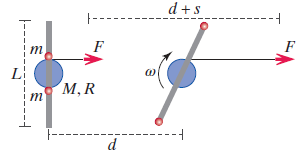A rod of length L and negligible mass is attached to a uniform disk of mass M and radius R (see figure below). A string is wrapped around the disk, and you pull on the string with a constant force F. Two small balls each of mass m slide along the rod with negligible friction. The apparatus starts from rest, and when the center of the disk has moved a distance d, a length of strings has come off the...

• ### A uniform solld disk of radius R and mass M is free to rotate on a...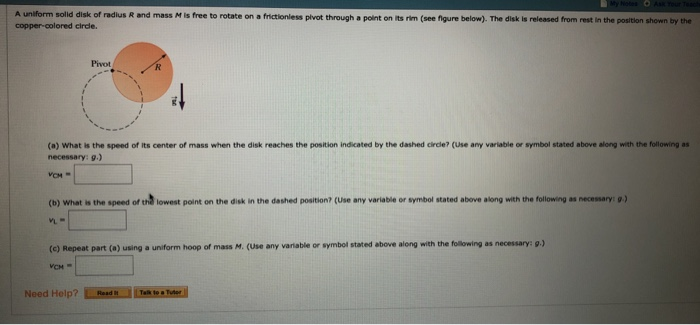A uniform solld disk of radius R and mass M is free to rotate on a frictionless plvot through a point on its rim (see figure below). The disk is released from rest In the position shown by the Pivot (a) What is the speed of its center of mass when the disk reaches the position indicated by the dashed circle? (Use any variable or symbol stated above along with the following as necessary: g) (b) What is the speed...